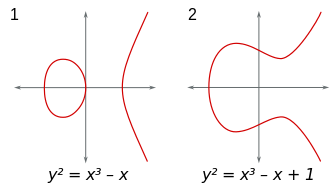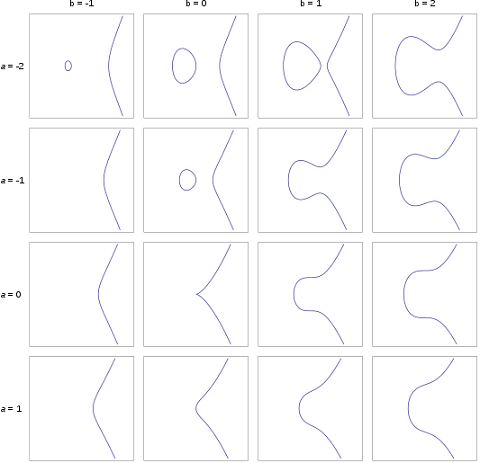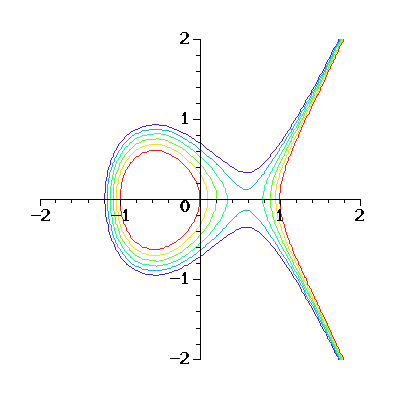﻿ ﻿
﻿

What Is an Elliptic Curve?

This section describes what is elliptic curve - A set of 2 dimensional points of (x, y) that satisfy the y*y = x*x*x + a*x + b equation with given values of a and b.

﻿

What Is an Elliptic Curve? - An elliptic curve is the set of 2 dimensional points of (x, y) that satisfy the following mathematical equation with given values of a and b:

```y2 = x3 + ax + b
```

Here are two examples of elliptic curves (source: wikipedia.org):Examples of Elliptic Curves

Here are more examples of elliptic curves (source: wikipedia.org):More Examples of Elliptic Curves ﻿

Here are some elliptic curves superposed like contour lines (source: cosec.bit.uni-bonn.de):Elliptic Curves Superposed Like Contour Lines

By the way, the earliest text book discussing elliptic curves is probably the "Arithmetica" by "the father of algebra", Diophantus of Alexandria in the third century.

﻿

﻿ ﻿

Table of Contents